# Conic Sections

Conic Sections are figures that can be formed by slicing a three dimensional right
circular cone with a plane. There are different ways to do this, and each way yields
a different figure. These figures can be represented on the graph as well as algebraically.
The four conic sections are circles, ellipses, parabolas, and hyperbolas.Conic Sections have been studied for a quite a long time. Kepler first noticed that
planets had elliptical orbits. Depending on the energy of an orbiting body, orbit
shapes that are any of the four types of conic sections are possible.

A conic section can be formally defined as a set or locus of a point that moves
in the plane of a fixed point called the focus and the fixed line is called
the directrix.

The general equation for all conics isand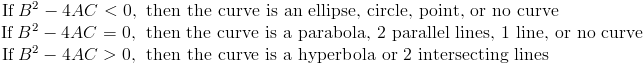## Circles

A circle is formed by cutting a circular cone with a plane perpendicular to the
symmetry axis of the cone. This intersection is a closed curve, and the intersection
is parallel to the plane generating the circle of the cone. A circle is also the
set of all points that are equally distant from the center.

The equation of a circle is defined aswhere (h,k) is the center of the circle and r is the radius.

(1) Graph the circle centered at (3,-2) with radius 4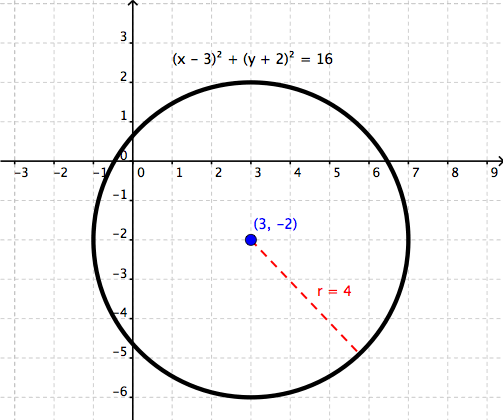In standard form, the equation would be## Ellipses

An ellipse is formed by cutting a three dimensional cone with a slanted plane. This
differs from a circle in that an ellipse does not have a constant radius. It has
two focal points in which the sum of the length of both focal points to any given
point on the ellipse is always the same.

The standard equation of an ellipse is given as follows.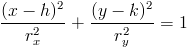where (h,k) is the center of the ellipse, rx is the distance from
the center of the circle in the x direction and ry
is the distance from the center in the y direction.

The foci of an ellipse is distance c, which is given by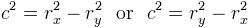from the center of the ellipse on the major axis. The major axis is the line
of the ellipse that has the biggest distance from the center of the circle. If the major axis is horizontal, 2rx
is the length and c2=rx2-ry2. If the major
axis is vertical, 2ry is the length and c2=ry2-rx2.where j+i = m+n = p+q. The sum of the distances from any point on the
ellipse to both foci will always be the same.

If the foci are close to the center, the ellipse will be closer to a circle. If
the foci are farther from the center, the ellipse will look more like an oval.

Let’s do an example.

(2) Graph the ellipse given by the equationWe first need to put it in standard form so we can find the x and y radius. We set
the right side equal to 1 by dividing both sides by 16.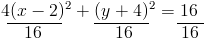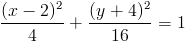Putting this in standard form, we would haveWe can see that the radius is (2,-4) and the x and y radii are 2 and 4 respectively.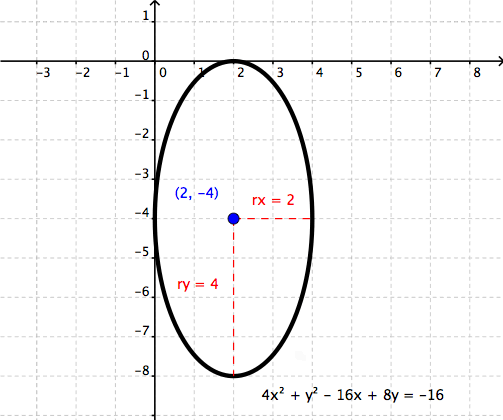Since y is the major axis, the foci will be determined by c2=ry2-rx2,
so c2=42-22 which yields the square root
of 10 for c. When we add and subtract c on the major axis from the
center, we get the foci.

## Parabolas

A parabola is formed by intersecting the plane through the cone and the top of the
cone. Parabolas can be the only conic sections that are considered functions because they pass the vertical line test.
We have worked with parabolas before in
, but parabolas formed by conic sections are a little
different.

A parabola is the set of points that are equally distant from a focus point and
the directrix, a fixed line. The standard equation depends on the axis of symmetry.
A vertical axis has a focus at (h,k+p) and the equation (x-h)2=4p(y-k).
A horizontal axis has a focus at (h+p,k) and the equation (y-k)2=4p(x-h).
The vertex is always halfway in between the focus and directrix at a distance p
from both.In this image, b = d, e = f, and g = h.

(3) Graph x2 = -16y and locate the focus and directrix.

By inspection, we can see that the x is squared so the parabola will either be opening
upwards or downwards. The value in front of y is negative so it must open downwards.
The vertex is also located at (0,0) and the length of the focus from the
vertex is 4. By going down from the vertex by 4, we can see that the focus is at
(0,-4) and the directrix will be at y = 4.## Hyperbolas

A hyperbola is formed when a plane slices the top and bottom section of the cone.
The equation for a hyperbola iswhere (h,k) is the center between the curves and it’s two asymptotes go through
the points (+a,-b) and (-a,+b) as well as (a,b) and (-a,-b)
starting at the center point.

(4) Graph the image of the parabola given the equationWe can see the center is (0,3) and the vertices of the parabolas formed will
be 2 left and right from the center. Once we have our diagonal asymptotes, we can
construct the hyperbola.Which forms the image of the hyperbola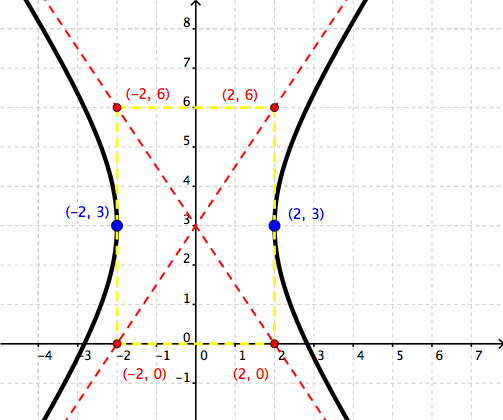## Tutoring

Looking for someone to help you with algebra? At Wyzant, connect with algebra tutors and math tutors nearby. Prefer to meet online? Find online algebra tutors or online math tutors in a couple of clicks.

Scroll to Top